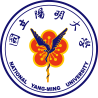National Yang Ming University

Equation11:

\frac{d[LacI]}{dt}={RBS}\times{[mRNA LacI]} - {Kdeg LacI} \times{[LacI]}

RBS = binding site strength

KdegLacI = degrading constant of LacI

The aim of the equation is to knowLacI production rate and when it can reach the concentration to repress promoter LacI.

'Equation12:

\frac{d[LuxI]}{dt}={RBS}\times{[mRNA LuxI]} -KAHL\times{[LuxI]} -Kdeg {[LuxI]} \times{[LuxI]}

RBS = binding site strength

KAHL = LuxI to AHL rate constant

KdegLuxI = degrading constant of LuxI

The aim of the equation is to know the production rate of LuxI concerning the LuxIAHL reaction and LuxI degrading.

'Equation13:

\frac{d[LuxR]}{dt}={RBS}\times{[mRNA LuxR]} – {KmAHL}\times{[AHL]} - {Kdeg LuxR} \times{[LuxR]} \times {KmAHL}\{times[AHL]^{2}} \times{[LuxR]}- KdegAHL\times{[AHL]}

RBS = binding site strength

KmAHL =AHL to LuxI rate constant

KonAHL = 2AHL + LuxR to (AHL)2/LuxR complex rate constant

KdegAHL = degrading constant of AHL

The aim of the equation is to know the production rate of LuxR concerning the 2AHL + LuxR to (AHL)2/LuxR reaction , AHL to LuxI reaction, and LuxR, AHL degrading.

'Equation18:

\frac{d[AHL]}{dt}=\times KonAHL \times [AHL]^{2}\times{[LuxR]}- {KoffAHL} \times {[AHLLuxR]} - KdegAHL\times{[AHL]}

KAHL = LuxI to AHL rate constant

KonAHL = 2AHL + LuxR to (AHL)2/LuxR complex rate constant

KoffAHL = (AHL)2/LuxR complex to 2AHL + LuxR rate constant

KdegAHL = degrading constant of AHL

The aim of the equation is to know the production rate of AHL concerning the LuxIAHL reaction, 2AHL + LuxR to (AHL)2/LuxR reaction, (AHL)2/LuxR complex to 2AHL + LuxR reaction and AHL degrading.